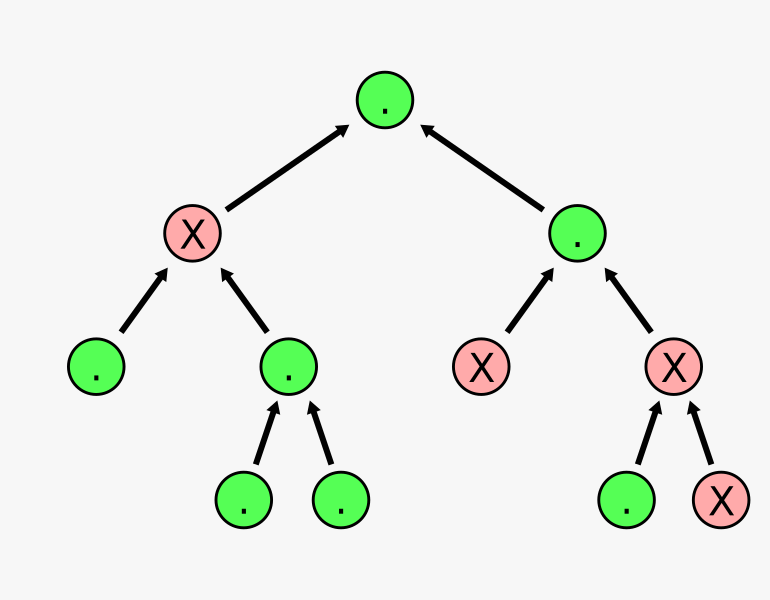# muhuk's blog

## Nature, to Be Commanded, Must Be Obeyed

September 01, 2015

# The Tree of Life: Walking the Tree

This is the third part of The Tree of Life series. You can read the first post here. In this post we will look into parsing paths and finding out the values of the nodes they target.

Let’s consider the tree in the example which is encoded as "((. X (. . .)) . (X . (. X X)))":We need a walk function that takes a tree and a path, and returns the value of the node the path points to. For the tree above it should produce the following outputs:

```walk(tree, "") -> '.'      // 1: Empty path points to root node.
walk(tree, ">") -> '.'     // 2: Go right.
walk(tree, ">>") -> 'X'    // 3: Go right, then go right again.
walk(tree, ">><") -> '.'   // 4: Go right, right and then left.
```

Here the resulting nodes are marked:

```((. X (. . .)) . (X . (. X X)))
|    |  | |
1    |  | |
2  | |
4 |
3
```

As you can see walk starts with the root node and walks down the tree according to the directions ('<' or '>').

Let’s start by writing a version of walk that only works right for a path of "":

```def walk(tree: TreeNode, path: List[Char]): Char = if (tree.value) 'X' else '.'
```

This is of course useless, but we need to start somewhere. Remember how be divided the problem into four parts in the first post. A complex task can be daunting at first. We should split it into smaller and smaller pieces until we have a number of feasible, perhaps even trivial tasks to deal with. And when their solutions are combined, we would have a solution to the initial task we found complex and difficult. This is similar. But the version of walk above is broken. We have started with a less demanding requirement to build our solution on, instead of breaking down a complex requirement. In other words I wouldn’t want anyone to see it, except momentarily on my screen while I’m refactoring it to a valid implementation. Now we refactor this to support "", "<" and ">":

```def walk(tree: TreeNode, path: List[Char]): Char = (tree, path) match {
case (_, Nil) => if (tree.value) 'X' else '.'
case (BranchNode(_, l, _), '<' :: Nil) => walk(l, Nil)
case (BranchNode(_, _, r), '>' :: Nil) => walk(r, Nil)
}
```

This version is better but it allows only one level of recursion. Rearranging it a little bit we can handle all valid inputs:

```def walk(tree: TreeNode, path: List[Char]): Char = (tree, path) match {
case (BranchNode(_, l, _), '<' :: rest) => walk(l, rest)
case (BranchNode(_, _, r), '>' :: rest) => walk(r, rest)
case (node, Nil) => if (node.value) 'X' else '.'
case _ => throw new RuntimeException()
}
```

What we do here is either to walk the left or right branch when we have a '<' or '>' at the head of our path. We go down the tree like this until the path is empty. Last case is added to get rid of the compiler warning about match not being exhaustive. You should never get a RuntimeException on valid inputs.

Notice that walk is tail recursive. Annotating it with scala.annotation.tailrec would cause the compiler to warn you if it doesn’t actually get optimized.

Next and last post will be about the top level code that uses what we’ve built so far.

If you have any questions, suggestions or corrections feel free to drop me a line.